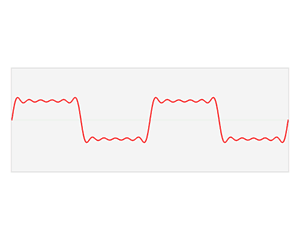# Frequency domainIn electronics, control systems engineering, and statistics, the frequency domain refers to the analysis of mathematical functions or signals with respect to frequency, rather than time. Put simply, a time-domain graph shows how a signal changes over time, whereas a frequency-domain graph shows how much of the signal lies within each given frequency band over a range of frequencies. A frequency-domain representation can also include information on the phase shift that must be applied to each sinusoid in order to be able to recombine the frequency components to recover the original time signal.

A given function or signal can be converted between the time and frequency domains with a pair of mathematical operators called a transform. An example is the Fourier transform, which converts the time function into a sum of sine waves of different frequencies, each of which represents a frequency component. The 'spectrum' of frequency components is the frequency domain representation of the signal. The inverse Fourier transform converts the frequency domain function back to a time function. A spectrum analyzer is the tool commonly used to visualize real-world signals in the frequency domain.

Some specialized signal processing techniques use transforms that result in a joint time-frequency domain, with the instantaneous frequency being a key link between the time domain and the frequency domain.

## Magnitude and phase

In using the Laplace, Z-, or Fourier transforms, a signal is described by a complex function of frequency: the component of the signal at any given frequency is given by a complex number. The magnitude of the number is the amplitude of that component, and the angle is the relative phase of the wave. For example, using the Fourier transform a sound wave, such as human speech, can be broken down into its component tones of different frequencies, each represented by a sine wave of a different amplitude and phase. The response of a system, as a function of frequency, can also be described by a complex function. In many applications, phase information is not important. By discarding the phase information it is possible to simplify the information in a frequency domain representation to generate a frequency spectrum or spectral density. A spectrum analyzer is a device that displays the spectrum, while the time domain frequency can be seen on an oscilloscope.

The power spectral density is a frequency-domain description that can be applied to a large class of signals that are neither periodic nor square-integrable; to have a power spectral density, a signal needs only to be the output of a wide-sense stationary random process.

## Different frequency domains

Although "the" frequency domain is spoken of in the singular, there are a number of different mathematical transforms which are used to analyze time domain functions and are referred to as "frequency domain" methods. These are the most common transforms, and the fields in which they are used:

More generally, one can speak of the transform domain with respect to any transform. The above transforms can be interpreted as capturing some form of frequency, and hence the transform domain is referred to as a frequency domain.

## Discrete frequency domain

The Fourier transform of a periodic signal only has energy at a base frequency and its harmonics. Another way of saying this is that a periodic signal can be analyzed using a discrete frequency domain. Dually, a discrete-time signal gives rise to a periodic frequency spectrum. Combining these two, if we start with a time signal which is both discrete and periodic, we get a frequency spectrum which is both periodic and discrete. This is the usual context for a discrete Fourier transform.

## History of term

The use of the terms "frequency domain" and "time domain" arose in communication engineering in the 1950s and early 1960s, with "frequency domain" appearing in 1953. See time domain: origin of term for details.